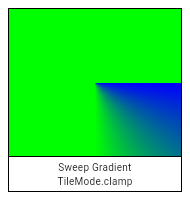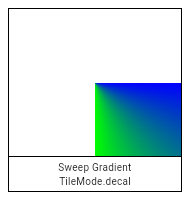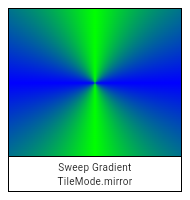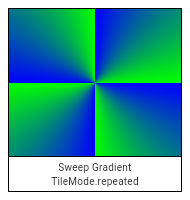1. Offset center,
2. colors,
3. [List<double>? colorStops,
4. TileMode tileMode = TileMode.clamp,
5. double startAngle = 0.0,
6. double endAngle = math.pi * 2,
7. matrix4]
)

Creates a sweep gradient centered at `center` that starts at `startAngle` and ends at `endAngle`.

`startAngle` and `endAngle` should be provided in radians, with zero radians being the horizontal line to the right of the `center` and with positive angles going clockwise around the `center`.

If `colorStops` is provided, `colorStops[i]` is a number from 0.0 to 1.0 that specifies where `color[i]` begins in the gradient. If `colorStops` is not provided, then only two stops, at 0.0 and 1.0, are implied (and `color` must therefore only have two entries).

The behavior before `startAngle` and after `endAngle` is described by the `tileMode` argument. For details, see the TileMode enum.If `center`, `colors`, `tileMode`, `startAngle`, or `endAngle` are null, or if `colors` or `colorStops` contain null values, this constructor will throw a NoSuchMethodError.

If `matrix4` is provided, the gradient fill will be transformed by the specified 4x4 matrix relative to the local coordinate system. `matrix4` must be a column-major matrix packed into a list of 16 values.

## Implementation

``````Gradient.sweep(
Offset center,
List<Color> colors, [
List<double>? colorStops,
TileMode tileMode = TileMode.clamp,
double startAngle = 0.0,
double endAngle = math.pi * 2,
Float64List? matrix4,
]) : assert(_offsetIsValid(center)),
assert(colors != null),
assert(tileMode != null),
assert(startAngle != null),
assert(endAngle != null),
assert(startAngle < endAngle),
assert(matrix4 == null || _matrix4IsValid(matrix4)),
super._() {
_validateColorStops(colors, colorStops);
final Int32List colorsBuffer = _encodeColorList(colors);
final Float32List? colorStopsBuffer = colorStops == null ? null : Float32List.fromList(colorStops);
_constructor();
_initSweep(center.dx, center.dy, colorsBuffer, colorStopsBuffer, tileMode.index, startAngle, endAngle, matrix4);
}``````